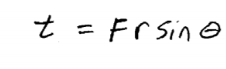Blog / Torques Lever Arms

### Torques, lever arms

Written by Chau Pham on Feb 26, 2020

For an object to be in equilibrium, it must be experiencing no acceleration. The second condition of static equilibrium says that the net torque acting on the object must be zero.

The second condition necessary to achieve equilibrium involves avoiding accelerated rotation. Torque is the rotational equivalent of a force. Force causes acceleration in translational motion. Torque causes acceleration in rotational motion: makes an object spin around a pivot. Torque is a measure of the effectiveness of a force in changing or accelerating a rotation (changing the angular velocity over a period of time).

Imagine you have a wrench that can turn a screw. The stronger you push on this wrench, the more you’re going to turn it.Also take into consideration where you apply the force, and where the rotation occurs. The location where the rotation occurs is the pivot point. The distance between the applied force and the pivot point is the lever arm. The longer the lever arm, the easier it is to turn the wrench.

Besides, the angle of which the force is applied is important. Pushing perpendicular to this lever arm will give you more torque than pushing parallel to it. In this case, the angle is 90 degrees.τ (the Greek letter tau) is the symbol for torque, r is the distance from the pivot point to the point where the force is applied, F is the magnitude of the force, and θ is the angle between the force and the vector directed from the point of application to the pivot point.

If the resultant moment about a particular axis is zero, the object will have no rotational acceleration about the axis. If the object is not spinning, it will not start to spin. If the object is spinning, it will continue to spin at the same constant angular

### KEY TAKEAWAYS

#### Key Points

• In equilibrium, it must be experiencing no acceleration. The second condition necessary to achieve equilibrium involves avoiding accelerated rotation.
• A rotating body or system can be in equilibrium if its rate of rotation is constant and remains unchanged by the forces acting on it.
• The magnitude of torque about a axis of rotation is defined to be τ=rFsinθ.

#### Key Terms

• Torque: A rotational or twisting effect of a force; (SI unit newton-meter or Nm; imperial unit foot-pound or ft-lb)
• Pivot point: The location where the rotation occurs
• Lever arm: distance between the applied force and the pivot point

Billing Information